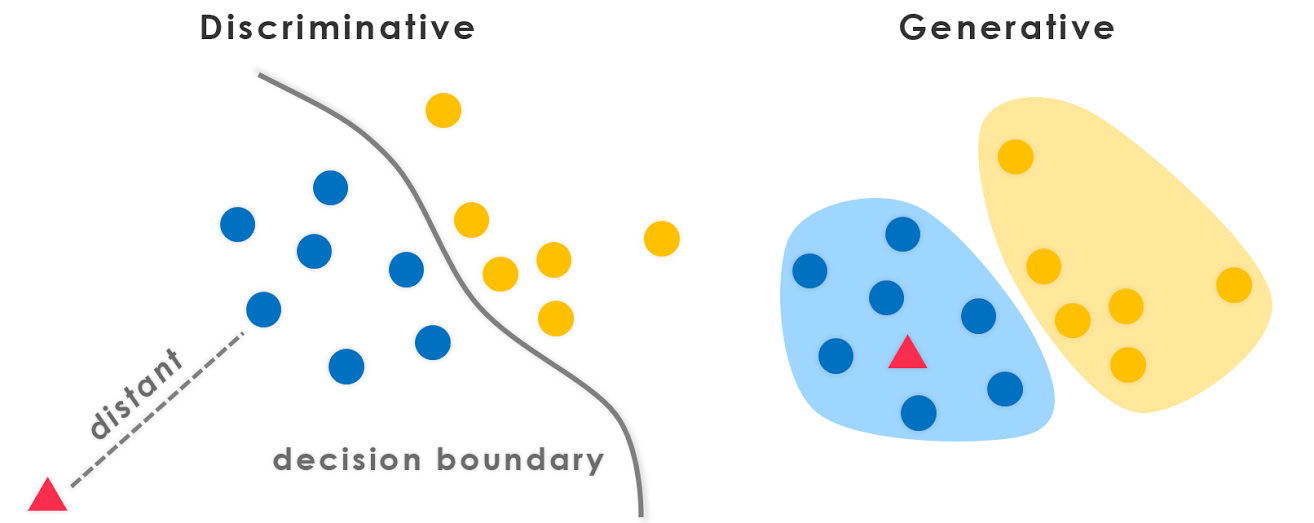/  Top Machine learning interview questions and answers   /  What is the differnce between Generative and Discrimination models?## What is the differnce between Generative and Discrimination models?

### Generative Model

A Generative Model ‌learns the joint probability distribution p (x, y). It predicts the conditional probability with the help of Bayes Theorem. A Generative Model ‌explicitly models the actual distribution of each class.

### Generative classifiers

• Assume some functional form for P(Y), P(X|Y)
• Estimate parameters of P(X|Y), P(Y) directly from training data
• Use Bayes rule to calculate P (Y |X)

### Generative classifiers examples

• ‌Naïve Bayes
• Bayesian networks
• Markov random fields
• ‌Hidden Markov Models (HMM)

### Discriminative Model

A Discriminative model ‌models the decision boundary between the classes. A Discriminative model ‌learns the conditional probability distribution p (y |x).

### Discriminative Classifiers

• Assume some functional form for P(Y|X)
• Estimate parameters of P(Y|X) directly from training data

### Discriminative Classifiers

• ‌Logistic regression
• Scalar Vector Machine
• ‌Traditional neural networks
• ‌Nearest neighbor
• Conditional Random Fields (CRF)s